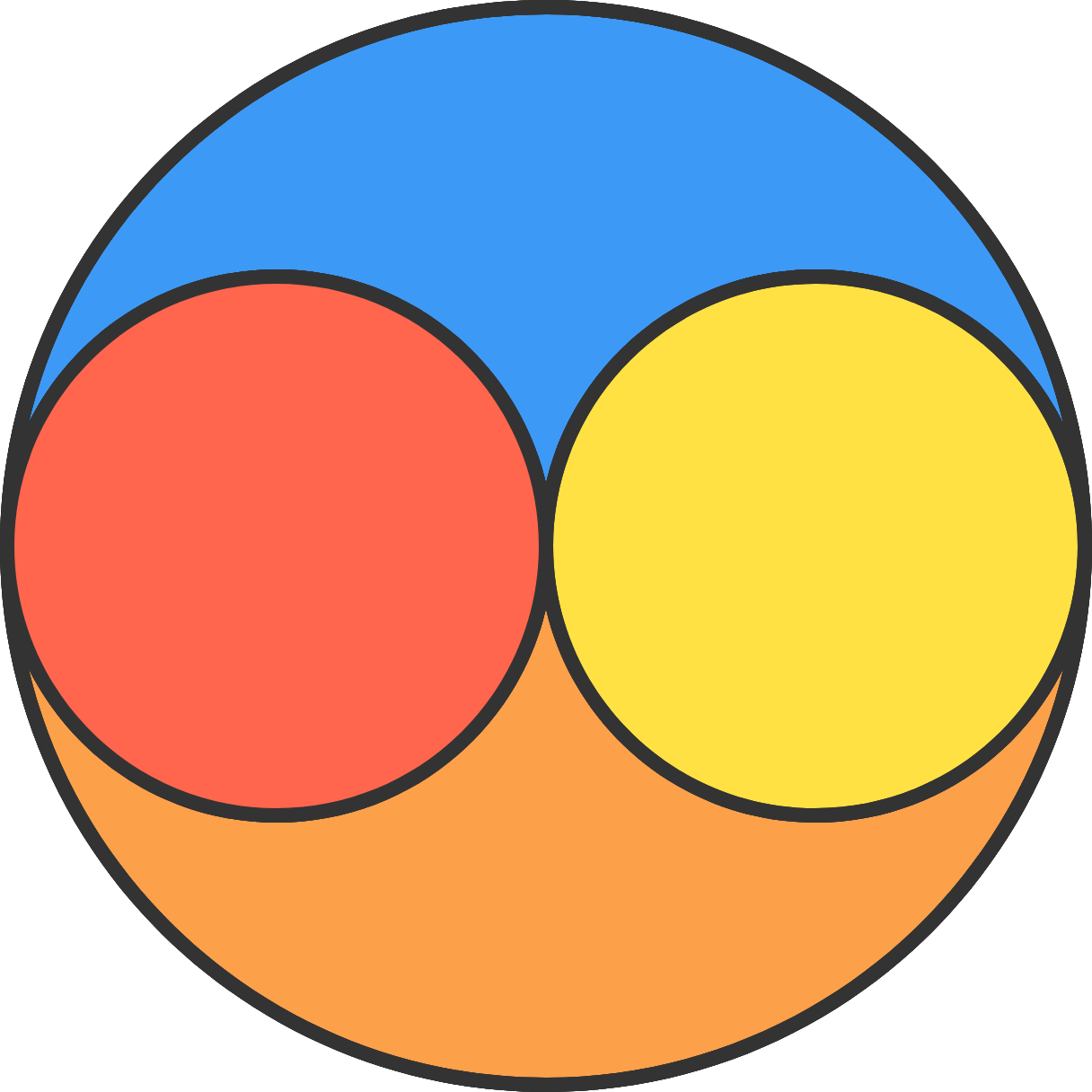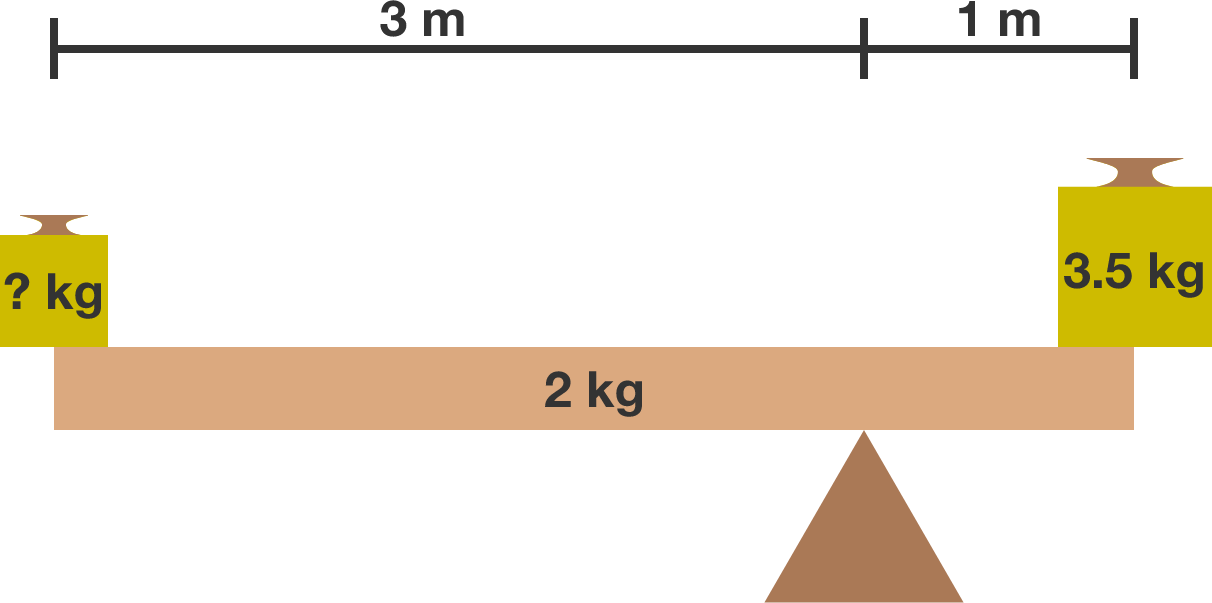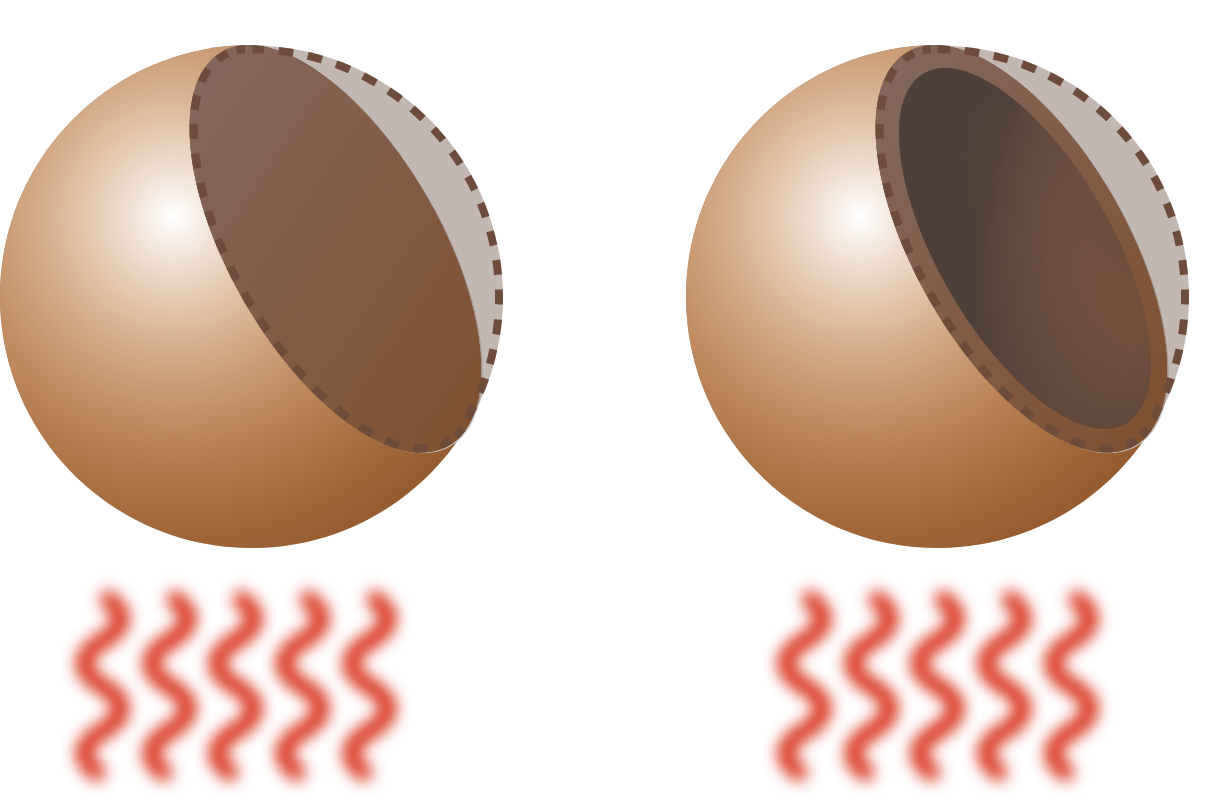# Problems of the Week

Contribute a problem

# 2017-10-30 Basic

\large \begin{aligned} 51 \times 51 &= {\color{#3D99F6}26}{\color{#D61F06}01} \\ 52 \times 52 &= {\color{#3D99F6}27}{\color{#D61F06}04} \\ 53 \times 53 &= {\color{#3D99F6}28}{\color{#D61F06}09} \\ 54 \times 54 &= {\color{#3D99F6}29}{\color{#D61F06}16} \\ 55 \times 55 &= {\color{#3D99F6}30}{\color{#D61F06}25} \\ 56 \times 56 &= {\color{#3D99F6}31}{\color{#D61F06}36} \\ & \vdots \end{aligned}

What is the value of $57 \times 57?$

Bonus: Can you explain why this pattern appears, and when it breaks?In the diagram, the yellow and red circles are identical and are both centered on the larger circle's diameter.

Which has a larger area?

In a 90-question, multiple-choice test, a student gets

• $+4$ for a correct answer
• $-1$ for an incorrect attempt.

If the student attempted every single question, can he or she get a total score of exactly 300?

For example, if the student only got 60 correct answers (30 incorrect), then the total score would be $60\times4 - 30\times1= 210.$

What mass (in kilograms) on the left side would keep the system in balance?We have two copper spheres of the same radius; one is hollow (vacuum inside) and the other is solid. Initially, both spheres are in a vacuum at the same temperature. Then they are heated up to the same temperature which causes them both to expand.

Which sphere has the greater final radius?×